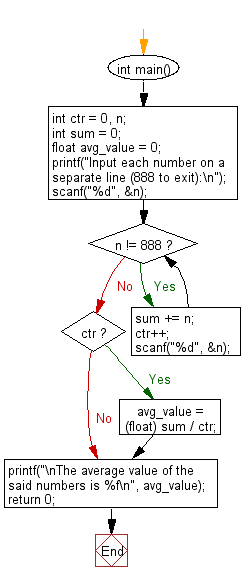﻿ C Program: Calculate and print the average of some integers - w3resource

# C Exercises: Calculate and print the average of some integers

## C Basic Declarations and Expressions: Exercise-84 with Solution

Write a C program to calculate and print the average of some integers. Accept all the values preceding 888.

Sample Input: 12
15
24
888

Sample Solution:

C Code:

``````#include <stdio.h>

int main()
{
int ctr = 0, n;       // Initialize counter and variable for input
int sum = 0;          // Initialize sum variable
float avg_value = 0;  // Initialize average variable

// Prompt user for input
printf("Input each number on a separate line (888 to exit):\n");

scanf("%d", &n);

// Loop until the user enters 888
while (n != 888) {
sum += n;  // Add the number to the sum
ctr++;     // Increment counter
scanf("%d", &n); // Read the next number
}

// Calculate the average if there were inputs
if (ctr)
avg_value = (float) sum / ctr;

// Print the average value
printf("\nThe average value of the said numbers is %f\n", avg_value);

return 0; // Indicate successful execution of the program
}
``````

Sample Output:

```Input each number on a separate line (888 to exit):

The average value of the said numbers is 17.000000
```

Flowchart:C programming Code Editor:

What is the difficulty level of this exercise?

Test your Programming skills with w3resource's quiz.

﻿